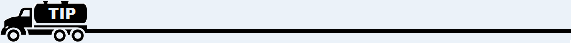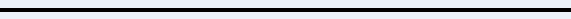﻿ COGS for Large Items

# Cost of Goods Sold for Large Items

At the end of each period (month), calculate the Cost Of Goods Sold for those items over \$100 (such as stoves and tanks). Since these tend to be low volume sales items with relatively fixed costs, you don't need to use the weighted average costing method that applies to propane.

1. For each large item category, find the number sold during the month from the Sales Summary.

2. Multiply this times the actual cost you paid for each item.

3. This is the Cost Of Goods Sold for each item.

Note: Left-click for an example of how this works.

4. When you've finished, print an Income Statement (report GL02 from General Ledger reports).  If the COGS account for each large item is zero, go to step 5 below. If it is not zero and there is a dollar amount in the COGS account, adjust (subtract) this amount from the Cost Of Goods Sold amount calculated above. Then go to step 5.

5. Key in a Journal Entry for the Cost of Goods Sold amount as a debit against the appropriate COGS account for each large item category in your General Ledger and a credit to the corresponding Inventory account.For further help regarding Cost Of Goods, go to:
Overview of Cost Of Goods Sold
Cost Of Goods Sold for Propane
Cost Of Goods Sold for Small Items